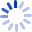Applied Regression Analysis, Linear Models, and Related Methods

by

An accessible, detailed, and up-to-date treatment of regression analysis, linear models, and closely related methods is provided in this book. Incorporating nearly 200 graphs and numerous examples and exercises that employ real data from the social sciences, the book begins with a consideration of the role of statistical data analysis in social research. It then moves on to cover the following topics: graphical methods for examining and transforming data; linear least-squares regression; dummy-variables regression; analysis ...

Cash for Textbooks